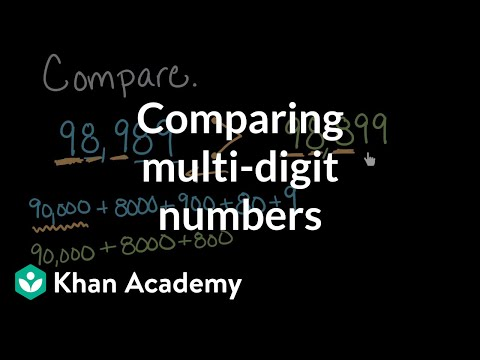Video

# Dividing whole numbers by 10 (Full video)

Description: Lindsay finds a pattern from dividing whole numbers by 10. So let's dig in and look at dividing by 10 and look at happens when we divide by 10 and see if we can figure out that pattern and maybe even how it relates to the pattern for multiplying by 10. One way to think about this is we're taking the number 30 and we're dividing it into groups of 10. So let's see how many groups of 10 it takes to get to 110.

### Other videos you might be interested in### Comparing multi-digit numbers (Full video)